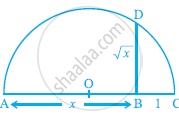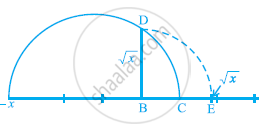# Operations on Real Numbers

#### notes

Rational numbers satisfy the commutative, associative and distributive laws for addition and multiplication. Moreover, if we add, subtract, multiply or divide (except by zero) two rational numbers, we still get a rational
number (that is, rational numbers are ‘closed’ with respect to addition, subtraction, multiplication and division). It turns out that irrational numbers also satisfy the commutative, associative and distributive laws for addition and multiplication. However, the sum, difference, quotients and products of irrational numbers are not always irrational. For example, (sqrt6)+(-sqrt6),(sqrt2)-(sqrt2),(sqrt3).(sqrt3) and sqrt7/sqrt7 are rationals.

Let us look at what happens when we add and multiply a rational number with an irrational number. For example, sqrt3 is irrational. What about 2 + sqrt3 and 2sqrt3? since sqrt3 has a non-terminating non-recurring decimal expansion, the same is true for 2+sqrt3 and 2sqrt3. Therefore, both 2 + sqrt3 and 2sqrt3 are also irrational numbers.

Let us see what generally happens if we add, subtract, multiply, divide, take square roots and even nth roots of these irrational numbers, where n is any natural number. Let us look at some examples.

Example : Add 2sqrt2+5sqrt3 and sqrt2-3sqrt3.

Solution: (2sqrt2+5sqrt3)+(sqrt2-3sqrt3)=(2sqrt2+sqrt2)+(5sqrt3-3sqrt3)

=(2+1)sqrt2+(5-3)sqrt3=3sqrt2+2sqrt3

Example: Mutiply 6sqrt5 by 2sqrt5.

Solution: 6sqrt5xx2sqrt5=6xx2xxsqrt5xxsqrt5=12xx5=60

Example: Divide 8sqrt(15) by 2sqrt3.

Solution: 8sqrt(15)÷2sqrt3=(8sqrt3xxsqrt5)/(2sqrt3)=4sqrt5

These examples may lead you to expect the following facts, which are true:
(i) The sum or difference of a rational number and an irrational number is irrational.
(ii) The product or quotient of a non-zero rational number with an irrational number is irrational.
(iii) If we add, subtract, multiply or divide two irrationals, the result may be rational or irrational.
We now turn our attention to the operation of taking square roots of real numbers.

Recall that, if a is a natural number, then sqrta=b means b= a and b>0.
The same definition can be extended for positive real numbers.

Let a > 0 be a real number. Then sqrta=b means b2 = a and b > 0.

In Section 1.2, we saw how to represent sqrtn for any positive integer n on the number line. We now show how to find sqrtx for any given positive real number x geometrically. For example, let us find it for x = 3.5, i.e., we find sqrt(3.5) geometrically.Fig. 1.15

Mark the distance 3.5 units from a fixed point A on a given line to obtain a point B such that AB = 3.5 units (see Fig. 1.15). From B, mark a distance of 1 unit and mark the new point as C. Find the mid-point of AC and mark that point as O. Draw a semicircle with centre O and radius OC. Draw a line perpendicular to AC passing through B and
intersecting the semicircle at D. Then, BD = sqrt(3.5).More generally, to find sqrtx,for any positive real number x, we mark B so that AB = x units, and, as in Fig. 1.16, mark C so that BC = 1 unit. Then, as we have done for the case x = 3.5, we find BD = sqrtx (see Fig. 1.16). We can prove this result using the Pythagoras Theorem.

Notice that, in Fig. 1.16, D OBD is a right-angled triangle. Also, the radius of the circle is (x+1)/2 units.

Therefore, OC = OD = OA = (x+1)/2 units.

Now, OB = x-((x+1)/2)=(x-1)/2.

So, by the Pythagoras Theorem, we have

BD^2=OD^2-OB^2=((x+1)/2)^2-((x-1)/2)^2=(4x)/4=x.

This shows that BD = sqrtx.

This construction gives us a visual, and geometric way of showing that sqrtx exists for all real numbers x > 0. If you want to know the position of sqrtx on the number line, then let us treat the line BC as the number line, with B as zero, C as 1, and so on. Draw an arc with centre B and radius BD, which intersects the number line in E
(see Fig. 1.17). Then, E represents sqrtx .Fig. 1.17

We would like to now extend the idea of square roots to cube roots, fourth roots, and in general nth roots, where n is a positive integer. Recall your understanding of square roots and cube roots from earlier classes.

What is root(3)(8) Well, we know it has to be some positive number whose cube is 8, and
you must have guessed root(3)(8)=2. Let us try root(5)(243). Do you know some number b such
that b5 = 243? The answer is 3. Therefore, root(5)(243) = 3

From these examples, can you define root(n)(a) for a real number a > 0 and a positive
integer n?

Let a > 0 be a real number and n be a positive integer. Then root(n)(a)= b, if bn = a and
b > 0. Note that the symbol 'sqrt' used in sqrt2, root(3)(8),root(n)(a), etc. is called the radical sign.

We now list some identities relating to square roots, which are useful in various ways. You are already familiar with some of these from your earlier classes. The remaining ones follow from the distributive law of multiplication over addition of real numbers, and from the identity (x + y) (x – y) = x2 – y2, for any real numbers x and y.
Let a and b be positive real numbers. Then

(i) sqrt(ab)=sqrta sqrtb

(ii) sqrt(a/b)=sqrta/sqrtb

(iii) (sqrta+sqrtb)(sqrta-sqrtb)=a - b

(iv) (a+sqrtb)(a-sqrtb)=a^2-b

(v) (sqrta+sqrtb)(sqrtc+sqrtd)=sqrt(ac)+sqrt(ad)+sqrt(bc)+sqrt(bd)

(vi) (sqrta+sqrtb)^2=a+2sqrt(ab)+b

Let us look at some particular cases of these identities.

Example : Simplify the following expressions:

(i) (5+sqrt7)(2+sqrt5)

(ii) (5+sqrt5)(5-sqrt5)

(iii) (sqrt3+sqrt7)^2

(iv) (sqrt(11)-sqrt7)(sqrt(11)+sqrt7)

Solution : (i) (5+sqrt7)(2+sqrt5)=10+5sqrt5+2sqrt7+sqrt(35)

(ii) (5+sqrt5)(5-sqrt5)=5^2-(sqrt5)^2=25-5=20

(iii) (sqrt3+sqrt7)^2(sqrt3)^2+2sqrt3 sqrt7+(sqrt7)^2=3+2sqrt(21)+7=10+2sqrt(21)

(iv) (sqrt(11)-sqrt7)(sqrt(11)+sqrt7)=(sqrt11)^2-(sqrt7)^2=11-7=4

Remark : Note that ‘simplify’ in the example above has been used to mean that the expression should be written as the sum of a rational and an irrational number.

We end this section by considering the following problem. Look at 1/sqrt2. Can you tell where it shows up on the number line? You know that it is irrational. May be it is easier to handle if the denominator is a rational number. Let us see, if we can ‘rationalise’ the denominator, that is, to make the denominator into a rational number. To do so, we
need the identities involving square roots. Let us see how.

Example : Rationalise the denominator of 1/sqrt2

Solution :We want to write 1/sqrt2 as an equivalent expression in which the denominator is a rational number. We know that sqrt2 . sqrt2 is rational. We also know that multiplying 1/sqrt2 by sqrt2/sqrt2 will give us an equivalent expression, since sqrt2/sqrt2 =1. So, we put these two facts together to get 1/sqrt2=1/sqrt2xxsqrt2/sqrt2=sqrt2/2.

In this form, it is easy to locate 1/sqrt2on the number line. It is half way between 0 and sqrt2 !

Example  : Rationalise the denominator of 1/2+sqrt3

Solution : We use the Identity (iv) given earlier. Multiply and divide 1/2+sqrt3 by 2-sqrt3 to get 1/(2+sqrt3)xx((2-sqrt3)/(2-sqrt3)=(2-sqrt3)/(4-3)=2-sqrt3

Example : Rationalise the denominator of 5/(sqrt3-sqrt5).

Solution : Here we use the Identity (iii) given earlier.

So, 5/(sqrt3-sqrt5)=5/(sqrt3-sqrt5)xx((sqrt3+sqrt5)/(sqrt3+sqrt5))=(5(sqrt3+sqrt5))/(3-5)=((-5)/2)(sqrt3+sqrt5)

Example : Rationalise the denominator of 1/(7+3sqrt2)

Solution: 1/(7+3sqrt2)=1/(7+3sqrt2)xx((7-3sqrt2)/(7-3sqrt2))=(7-3sqrt2)/(49-18)=(7-3sqrt2)/31

So, when the denominator of an expression contains a term with a square root (or a number under a radical sign), the process of converting it to an equivalent expression whose denominator is a rational number is called rationalising the denominator.

If you would like to contribute notes or other learning material, please submit them using the button below.

### Shaalaa.com

Operations on Real Number [00:06:01]
S
0%